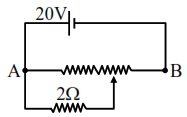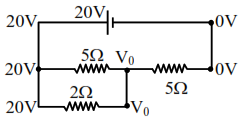# The given potentiometer

Question:

The given potentiometer has its wire of resistance $10 \Omega$. When the sliding contact is in the middle of the potentiometer wire, the potential drop across $2 \Omega$ resistor is :1. $10 \mathrm{~V}$

2. $5 \mathrm{~V}$

3. $\frac{40}{9} \mathrm{~V}$

4. $\frac{40}{11} \mathrm{~V}$

Correct Option: , 3

Solution:$\frac{20-\mathrm{V}_{0}}{5}+\frac{0-\mathrm{V}_{0}}{5}+\frac{20-\mathrm{V}_{0}}{2}=0$

$4+10=\frac{2 \mathrm{~V}_{0}}{5}+\frac{\mathrm{V}_{0}}{2}$

$14=\frac{4 \mathrm{~V}_{0}+5 \mathrm{~V}_{0}}{10}$

$\mathrm{V}_{0}=\frac{140}{9}$ Volt

Potential difference across $2 \Omega$ resistor is $20-\mathrm{V}_{0}$

That is $\left(20-\frac{140}{9}\right)$ Volt

Hence answer is $\left(\frac{40}{9}\right)$ Volt